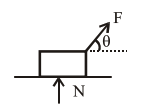Deepak Scored 45->99%ile with Bounce Back Crack Course. You can do it too!

# A body of mass

Question:

A body of mass $1 \mathrm{~kg}$ rests on a horizontal floor with which it has a coefficient of static friction $\frac{1}{\sqrt{3}}$. It is desired to make the body move by applying the minimum possible force $F \mathrm{~N}$. The value of $F$ will be __________ (Round off to the Nearest Integer)

[Take $\mathrm{g}=10 \mathrm{~ms}^{-2}$ ]

Solution:$\mathrm{F} \cos \theta=\mu \mathrm{N}$

$\mathrm{F} \sin \theta+\mathrm{N}=\mathrm{mg}$

$\Rightarrow \mathrm{F}=\frac{\mu \mathrm{mg}}{\cos \theta+\mu \sin \theta}$

$\mathrm{F}_{\min }=\frac{\mu \mathrm{mg}}{\sqrt{1+\mu^{2}}}=\frac{\frac{1}{\sqrt{3}} \times 10}{\frac{2}{\sqrt{3}}}=5$